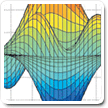Cody

# Problem 886. Rubik's Cube: Solve Randomized Cube - Score : Moves

The Challenge is to Solve a thoroughly scrambled Rubik's Cube.

The Performance metric is average number of moves for solving 200 cubes.

A standard Rubik's Cube is shown in 3-D and also unfolded to identify the specific Tile-face/Vector numbering.

The faces are White-Up / Red-Left / Blue-Front / Orange-Right / Yellow-Down / Green-Back (ULFRDB)

Moves are denoted as F for clockwise rotation of the Front face. F'(or FP) is CCW and F2 is F twice. The loaded function r_new=rubick_rot(mov,r) implements moves 1-18: UFDLBRU'F'D'L'B'R'U2F2D2L2B2R2.```Input: (rubik)
```
```rubik: row vector of size 54
(The cube started [L=0,U=1,F=2,D=3,B=4,R=5] and then receives forty face moves.
```
```Output: move_vec (Numeric of moves to solve)
move_vec:values 1:27 for UFDLBR U'F'D'L'B'R' U2F2D2L2B2R2 XYZX'Y'Z'X2Y2Z2
```
• Example:
• If the cube was randomized by [1 9] UD', one solution is [3 7] DU' which are the complements in reverse order.
• Scoring is average face moves for 200 cubes
• Cube Moves X, Y, and Z do not constitute a move but are needed in the vector
• A string to numeric value function is provided in the template
• Verifications will be by executing your move vector against the provided Rubik and counting number of face moves.

The function rubik_rot(mov,r) is available for intermediate processing

The Function Template Contains a 3D Cube Viewer and the first part of the Beginner's Solution at the bottom in a comment block.

Coming Soon: The Mini-Cube (2x2x2) Not as easy as it looks

### Solution Stats

5.56% Correct | 94.44% Incorrect
Last Solution submitted on Mar 06, 2020